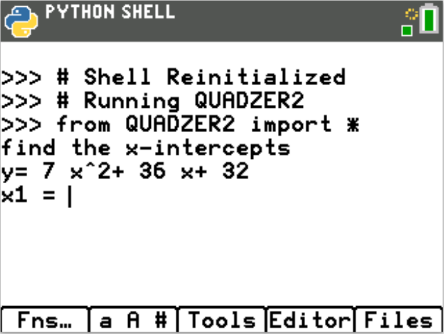Activities

•6-8
9-12

• Device
•TI-84 Plus CE Python
• Report an IssueActivity Overview

In this coding activity for the TI-84 Plus CE Python edition, students will extend the code from Integer Quadratic Zeros to create a game that allows exploration of other factored equation forms.

Objectives

• Use the input function and a variable to collect and store data from a user
• Use the randint() function to generate random integers.
• Use a while loop to repeat code
• Use if..elif..else statements to make decisions.
• Explore how x-intercepts are related to factored quadratic equations
• Explore how to factor equation in standard form
• Factor quadratic equations with rational solutions